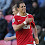# MATLAB Program for Solution Power Flow Gauss-Seidel Method | EE1404 – Power System Simulation Laboratory

AIM:
To understand, in particular, the mathematical formulation of power flow model in complex
form and a simple method of solving power flow problems of small sized system using Gauss-
Seidel iterative algorithm.

SOFTWARE REQUIRED:
MATLAB 5.3

THEORY:
The GAUSS – SEIDEL method is an iterative algorithm for solving a set of non-linear load
flow equations.

PROCEDURE:
1. Enter the command window of the MATLAB.
2. Create a new M – file by selecting File - New – M – File
3. Type and save the program in the
editor Window
4. Execute the program by either pressing Tools – Run.
5. View the results.

EXERCISE:
The figure shows the single line diagram of a simple 3 buses power system with generator at
bus 1.The magnitude at bus 1 is adjusted to 1.05pu. The scheduled loads at buses 2 and 3 are
marked on the diagram. Line impedance are marked in pu. The base value is 100kVA. The line
charging susceptances are neglected. Determine the phasor values of the voltage at the load
buses 2 and 3. Find the slack bus real and reactive power.
Verify the result using MATLAB.

PROGRAM:
%Gauss Sedial
clc;
data=[1 1 2 10-j*20
2 1 3 10-j*30
3 2 3 16-j*32]
elements=max(data(:,1));
bus=max(max(data(:,2)),max(data(:,3)));
y=zeros(bus,bus);
for p=1:bus,
for q=1:elements,
if(data(q,2)==p|data(q,3)==p)
y(p,p)=y(p,p)+data(q,4);
end
end
end
for p=1:bus,
for q=1:bus,
if (p~=q)
for r=1:elements
if((data(r,2)==p&data(r,3)==q)|(data(r,2)==q&data(r,3)==p))
y(p,q)=-(data(r,4));
end
end
end
end
end
a1=input('enter p2 in MW:');
b1=input('enter q2 in MVAR:');
a2=input('enter p3 in MW:');
b2=input('enter q3 in MVAR');
pu=input('enter the base value in MVA');
p2=(a1/pu);
q2=(b1/pu);
p3=(a2/pu);
q3=(b2/pu);
dx1=1+j*0;
dx2=1+j*0;
v1=1.05;
v2=1+j*0;
v3=1+j*0;
iter=0;
disp('iter v2 v3');
while(abs(dx1)&abs(dx2)>=0.00001)&iter<7;
iter=iter+1;
g1=(((p2-j*q2)/conj(v2))+(-y(1,2)*v1)+(-y(2,3)*v3))/y(2,2);
g2=(((p3-j*q3)/conj(v3))+(-y(1,3)*v1)+(-y(2,3)*g1))/y(3,3);
dx1=g1-v2;
dx2=g2-v3;
v2=v2+dx1;
v3=v3+dx2;
fprintf ('%g',iter),disp([v2,v3]);
end

RESULT:
Thus the mathematical formulation of power flow model in complex
form and a simple method of solving power flow problems of small sized system understood using Gauss-
Seidel iterative algorithm.
Previous
Next Post »

Why did you use 4*4 matrices if you have a three bus bar systemUnknown
AUTHOR

Where is the diagram ?Unknown
AUTHOR

Where is the Diagram ?